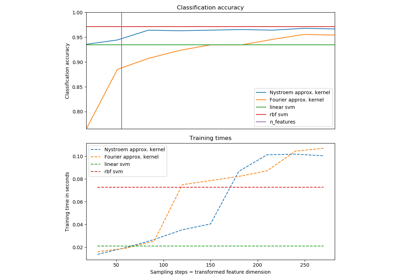# sklearn.kernel_approximation.RBFSampler¶

class sklearn.kernel_approximation.RBFSampler(gamma=1.0, n_components=100, random_state=None)[source]

Approximates feature map of an RBF kernel by Monte Carlo approximation of its Fourier transform.

It implements a variant of Random Kitchen Sinks.

Read more in the User Guide.

Parameters: gamma : float Parameter of RBF kernel: exp(-gamma * x^2) n_components : int Number of Monte Carlo samples per original feature. Equals the dimensionality of the computed feature space. random_state : int, RandomState instance or None, optional (default=None) If int, random_state is the seed used by the random number generator; If RandomState instance, random_state is the random number generator; If None, the random number generator is the RandomState instance used by np.random.

Notes

See “Random Features for Large-Scale Kernel Machines” by A. Rahimi and Benjamin Recht.

 “Weighted Sums of Random Kitchen Sinks: Replacing minimization with randomization in learning” by A. Rahimi and Benjamin Recht. (http://people.eecs.berkeley.edu/~brecht/papers/08.rah.rec.nips.pdf)

Examples

>>> from sklearn.kernel_approximation import RBFSampler
>>> from sklearn.linear_model import SGDClassifier
>>> X = [[0, 0], [1, 1], [1, 0], [0, 1]]
>>> y = [0, 0, 1, 1]
>>> rbf_feature = RBFSampler(gamma=1, random_state=1)
>>> X_features = rbf_feature.fit_transform(X)
>>> clf = SGDClassifier(max_iter=5, tol=1e-3)
>>> clf.fit(X_features, y)
...
SGDClassifier(alpha=0.0001, average=False, class_weight=None,
early_stopping=False, epsilon=0.1, eta0=0.0, fit_intercept=True,
l1_ratio=0.15, learning_rate='optimal', loss='hinge', max_iter=5,
n_iter=None, n_iter_no_change=5, n_jobs=None, penalty='l2',
power_t=0.5, random_state=None, shuffle=True, tol=0.001,
validation_fraction=0.1, verbose=0, warm_start=False)
>>> clf.score(X_features, y)
1.0


Methods

 fit(X[, y]) Fit the model with X. fit_transform(X[, y]) Fit to data, then transform it. get_params([deep]) Get parameters for this estimator. set_params(**params) Set the parameters of this estimator. transform(X) Apply the approximate feature map to X.
__init__(gamma=1.0, n_components=100, random_state=None)[source]
fit(X, y=None)[source]

Fit the model with X.

Samples random projection according to n_features.

Parameters: X : {array-like, sparse matrix}, shape (n_samples, n_features) Training data, where n_samples in the number of samples and n_features is the number of features. self : object Returns the transformer.
fit_transform(X, y=None, **fit_params)[source]

Fit to data, then transform it.

Fits transformer to X and y with optional parameters fit_params and returns a transformed version of X.

Parameters: X : numpy array of shape [n_samples, n_features] Training set. y : numpy array of shape [n_samples] Target values. X_new : numpy array of shape [n_samples, n_features_new] Transformed array.
get_params(deep=True)[source]

Get parameters for this estimator.

Parameters: deep : boolean, optional If True, will return the parameters for this estimator and contained subobjects that are estimators. params : mapping of string to any Parameter names mapped to their values.
set_params(**params)[source]

Set the parameters of this estimator.

The method works on simple estimators as well as on nested objects (such as pipelines). The latter have parameters of the form <component>__<parameter> so that it’s possible to update each component of a nested object.

Returns: self
transform(X)[source]

Apply the approximate feature map to X.

Parameters: X : {array-like, sparse matrix}, shape (n_samples, n_features) New data, where n_samples in the number of samples and n_features is the number of features. X_new : array-like, shape (n_samples, n_components)

## Examples using sklearn.kernel_approximation.RBFSampler¶Explicit feature map approximation for RBF kernels